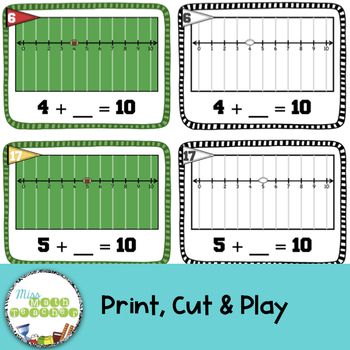# First Down: Make 10 Addition Football ThemedSubject
Resource Type
File Type

PDF

(8 MB|47 pages)
Standards
• Product Description
• StandardsNEW

Football is full of mathy goodness! Since I am ALWAYS searching for more ways to practice Make 10 addition facts, I could not wait to put together these cards to help my students get in some extra practice as we get psyched up for our Annual Math Super Bowl!

Cards can be used in many different ways. I created versions with and without numbers to make the more versatile. Check out the included sheet of activity ideas to get you started.

Set Includes:

• 22 Make 10 Addition cards with the equation
• Numbered card set in color
• Numbered card set in black/white
• Not numbered card set in color
• Not numbered card set in black/white

• 11 Make 10 Addition cards without equation
• Numbered card set in color
• Numbered card set in black/white
• Not numbered card set in color
• Not numbered card set in black/white

• Matching each set of cards
• Numbered sheets
• Not numbered sheets
• Colored and black/white of each

• Ideas for use
Fluently add and subtract within 20 using mental strategies. By end of Grade 2, know from memory all sums of two one-digit numbers.
Add and subtract within 20, demonstrating fluency for addition and subtraction within 10. Use strategies such as counting on; making ten (e.g., 8 + 6 = 8 + 2 + 4 = 10 + 4 = 14); decomposing a number leading to a ten (e.g., 13 - 4 = 13 - 3 - 1 = 10 - 1 = 9); using the relationship between addition and subtraction (e.g., knowing that 8 + 4 = 12, one knows 12 - 8 = 4); and creating equivalent but easier or known sums (e.g., adding 6 + 7 by creating the known equivalent 6 + 6 + 1 = 12 + 1 = 13).
Fluently add and subtract within 5.
For any number from 1 to 9, find the number that makes 10 when added to the given number, e.g., by using objects or drawings, and record the answer with a drawing or equation.
Decompose numbers less than or equal to 10 into pairs in more than one way, e.g., by using objects or drawings, and record each decomposition by a drawing or equation (e.g., 5 = 2 + 3 and 5 = 4 + 1).
Total Pages
47 pages
N/A
Teaching Duration
N/A
Report this Resource to TpT
Reported resources will be reviewed by our team. Report this resource to let us know if this resource violates TpT’s content guidelines.